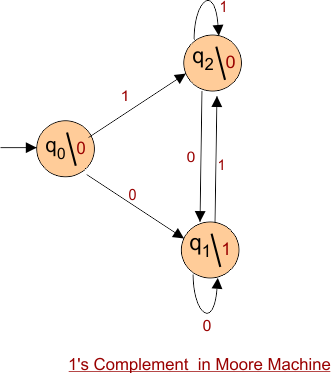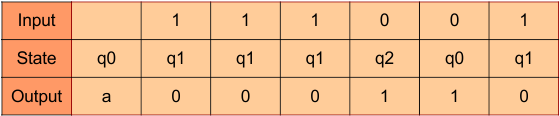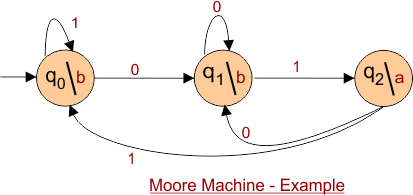Select Page

# Example of Automata Moore Machine

As we already covered the topic of Moore Machine. In this session we will see some examples of Automata Moore Machine.

## Example 1

Design a Moore machine to generate 1’s complement of a given binary number.

### Solution

According to first complement, if the given input is “1” then the output will be “0” and if the input is “0” then the output will be “1”.

For First complement there should be at least three states.

• One state is start state.
• The 2nd state is to accept “1’s” as input and produces output as “0”.
• The 3rd state will accept “0’s” as input and producing output as “1”.

Hence the final Moore machine of given example 1 will be,For instance, take one binary number 111001 thenThus we get 0000110 as 1’s complement of 111001, we can neglect the initial 0 and the output which we get is 000110 which is 1’s complement of 111001.

Important :The output for a Moore machine is greater than input in length by 1. Because start state always gives an output without consuming an input.

### Transition table

The Transition table of above constructed Moore Machine is given belowThus Moore machine 6 tuples {Q, q0, ∑, O, δ, λ} are explained below where

• Q = {q0, q1, q2},
• q0 is the initial state
• ∑ = {0, 1},
• O = {0, 1}. the transition table shows the
• Remaining two tuples ( δ and λ functions) are shown in the transition table

## Example 02

Construct a Automata Moore Machine that prints “a” whenever the sequence “01” is encountered in any input binary string.

### Solution

Automata Moore Machine for given example is given belowHelp Other’s By Sharing…